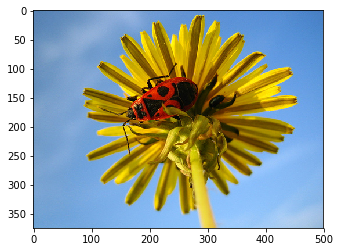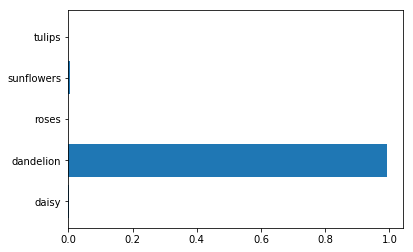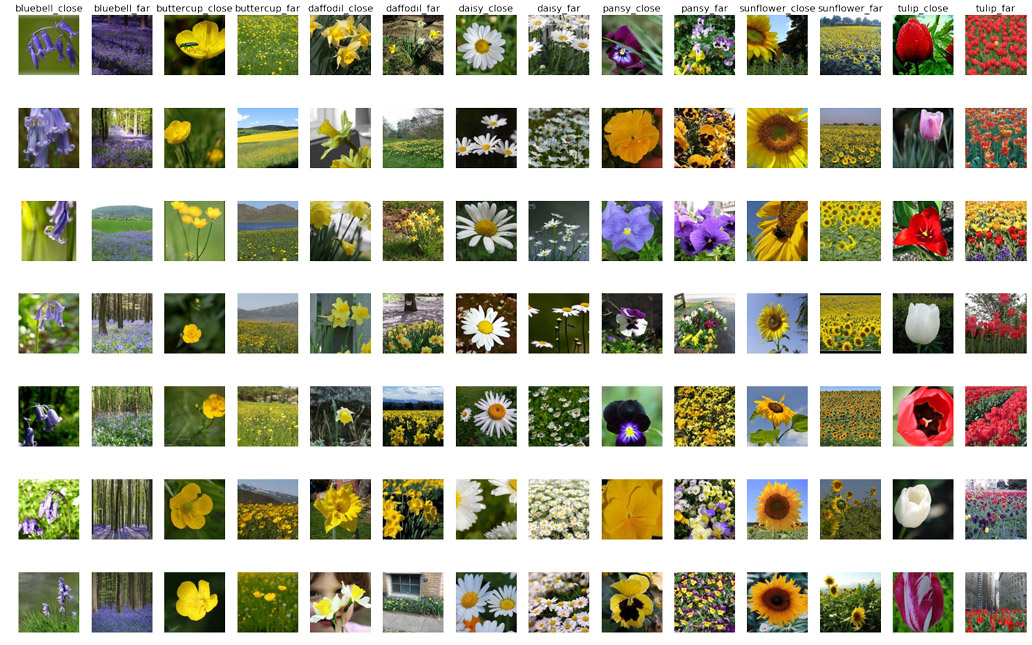# 什么是迁移学习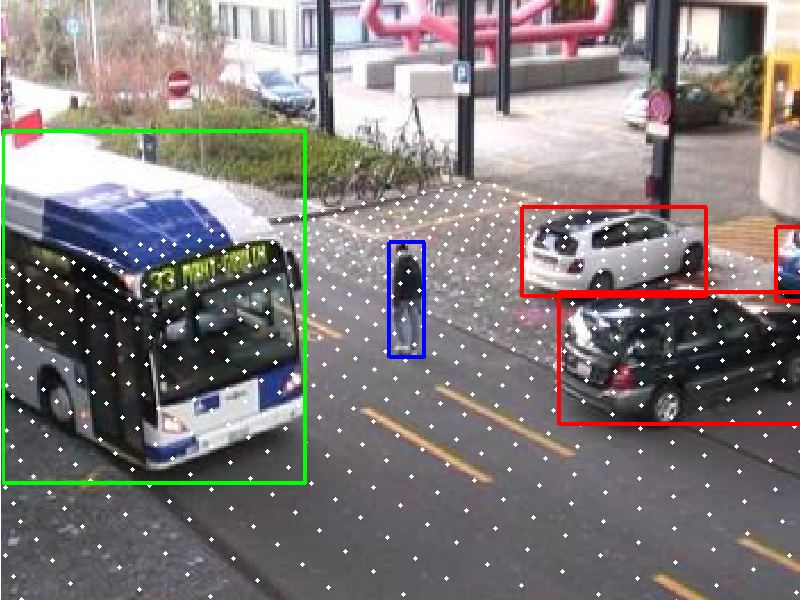# VGG介绍

VGG是视觉领域竞赛ILSVRC在2014年的获胜模型，以7.3%的错误率在ImageNet数据集上大幅刷新了前一年11.7%的世界纪录。VGG16基本上继承了AlexNet的思想，并且发扬光大，做到了更深。AlexNet只用到了8层网络，而VGG的两个版本分别是16层网络版和19层网络版。在接下来的迁移学习实践中，我们会采用稍微简单的一些的VGG16，他和VGG19有几乎完全一样的准确度，但是运算起来更快一些。

VGG的结构图如下：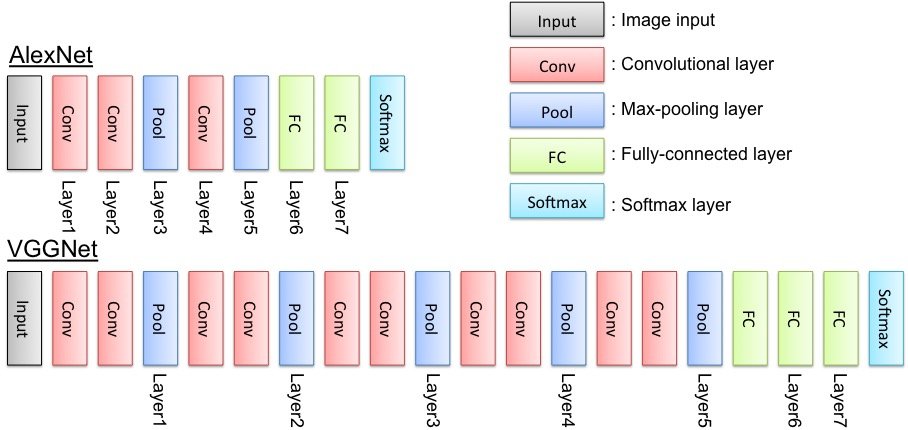VGG的输入数据格式是244 * 224 * 3的像素数据，经过一系列的卷积神经网络和池化网络处理之后，输出的是一个4096维的特征数据，然后再通过3层全连接的神经网络处理，最终由softmax规范化得到分类结果。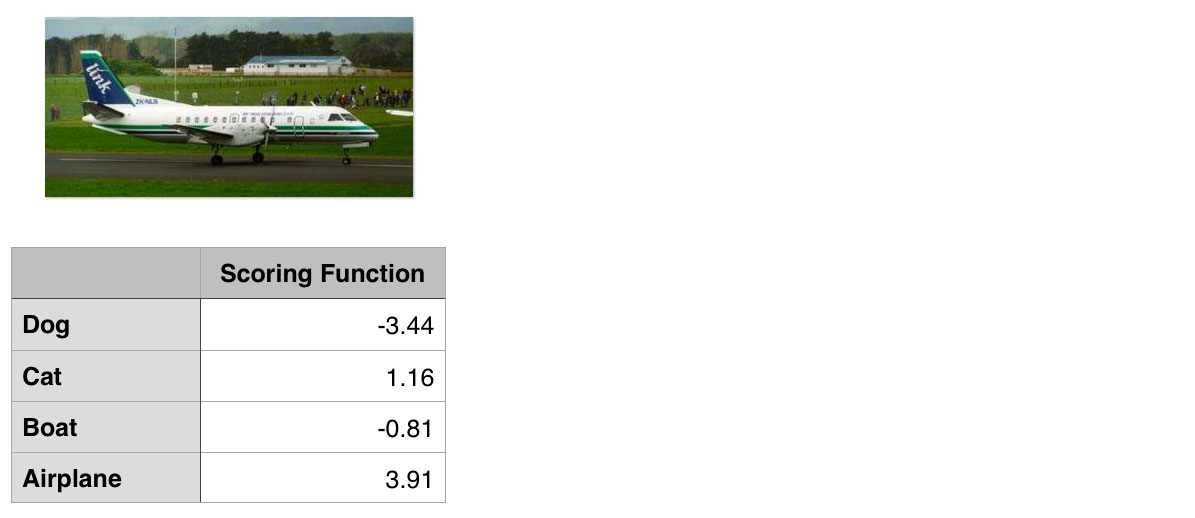VGG16模型可以通过这里下载（密码78g9），模型是一个.npy文件，本质上是一个巨大的numpy对象，包含了VGG16模型中的所有参数，该文件大约有500M，所以可见如果是从头训练这样一个模型是非常耗时的，借助于迁移学习的思想，我们可以直接在这个模型的基础上进行训练。

# 卷积神经网络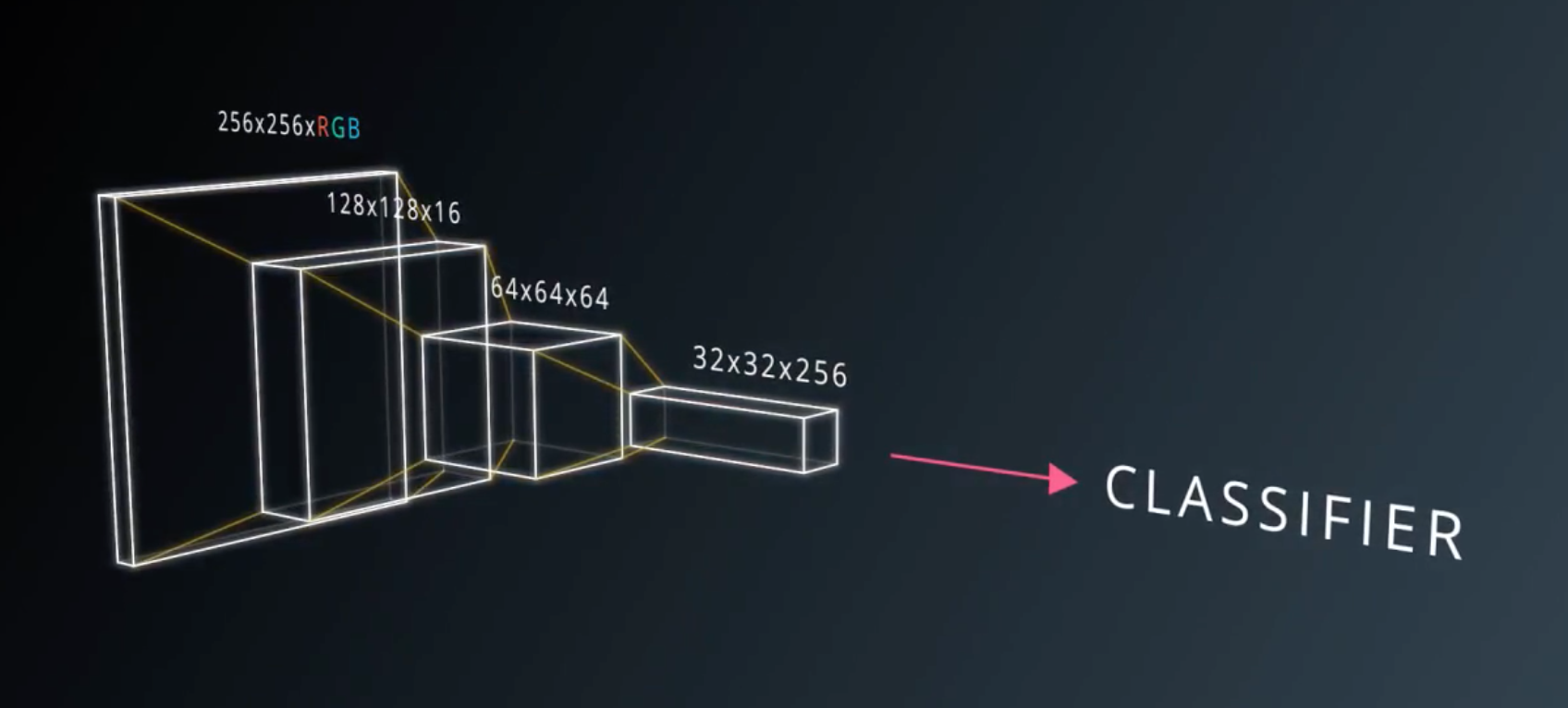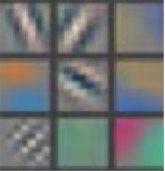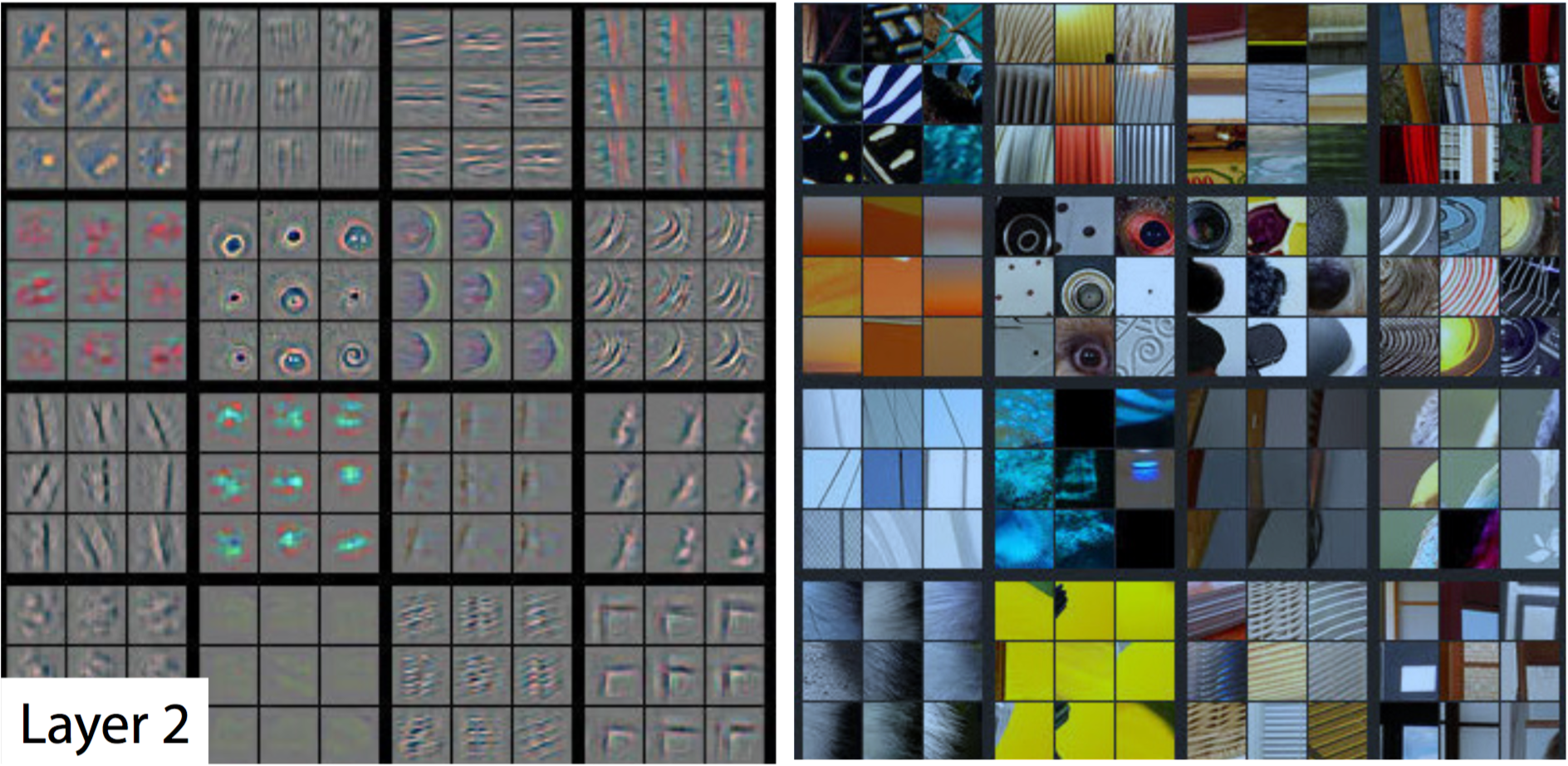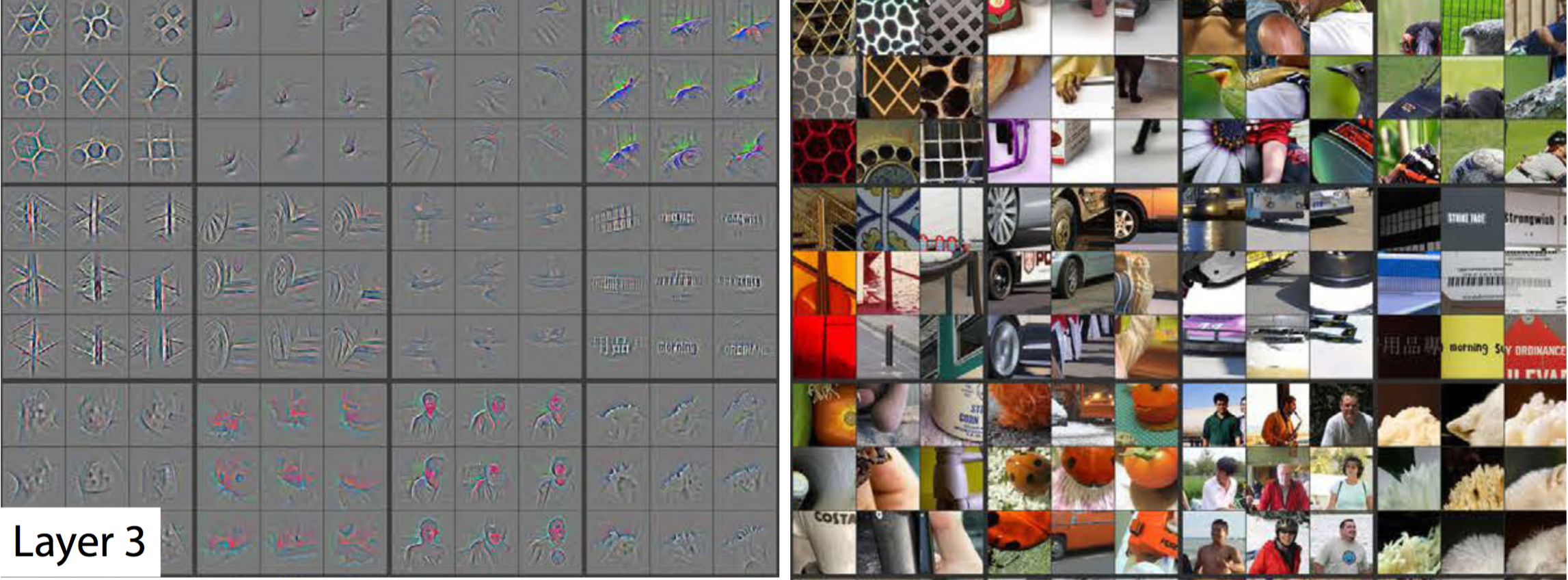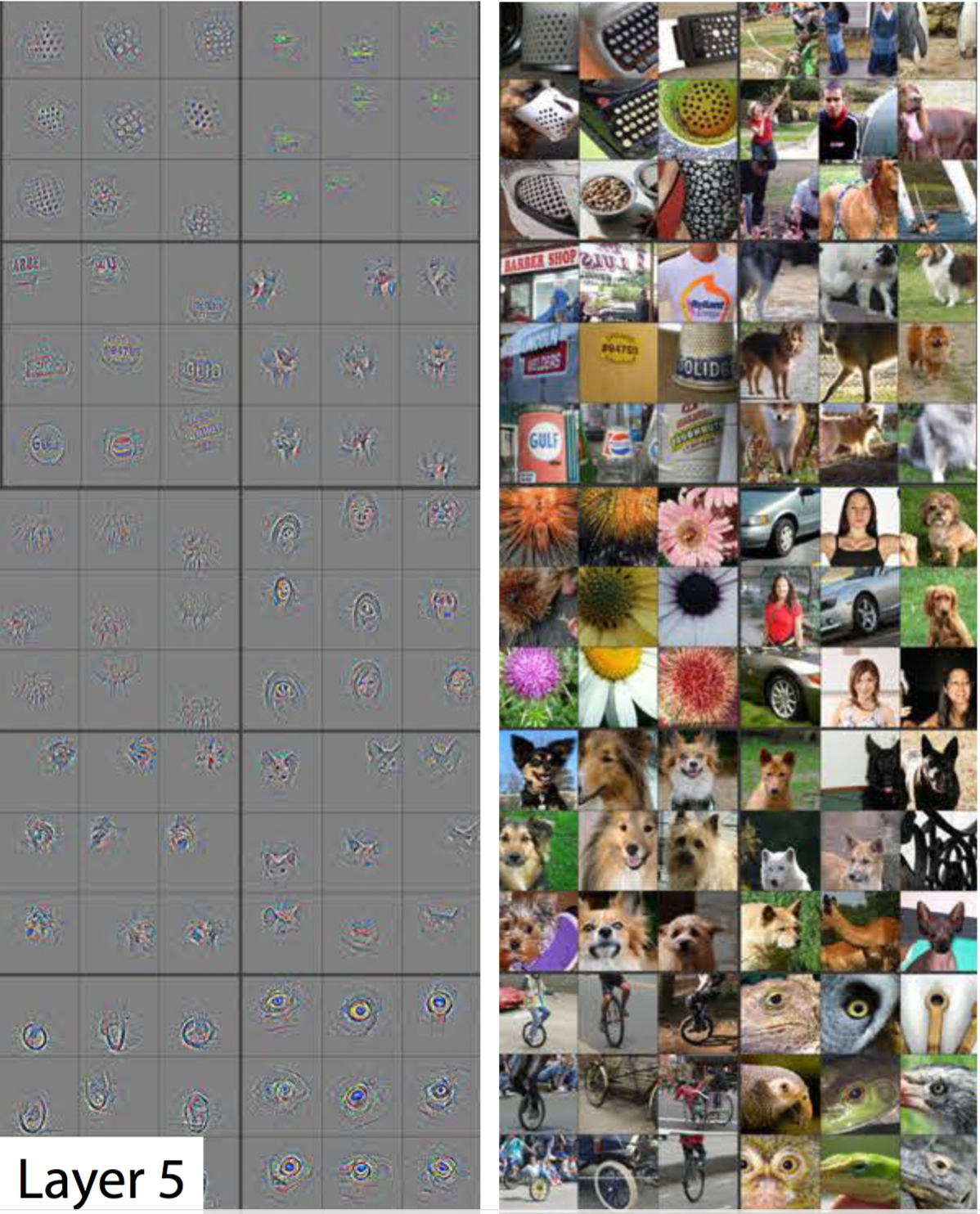# Max Pooling和Drop out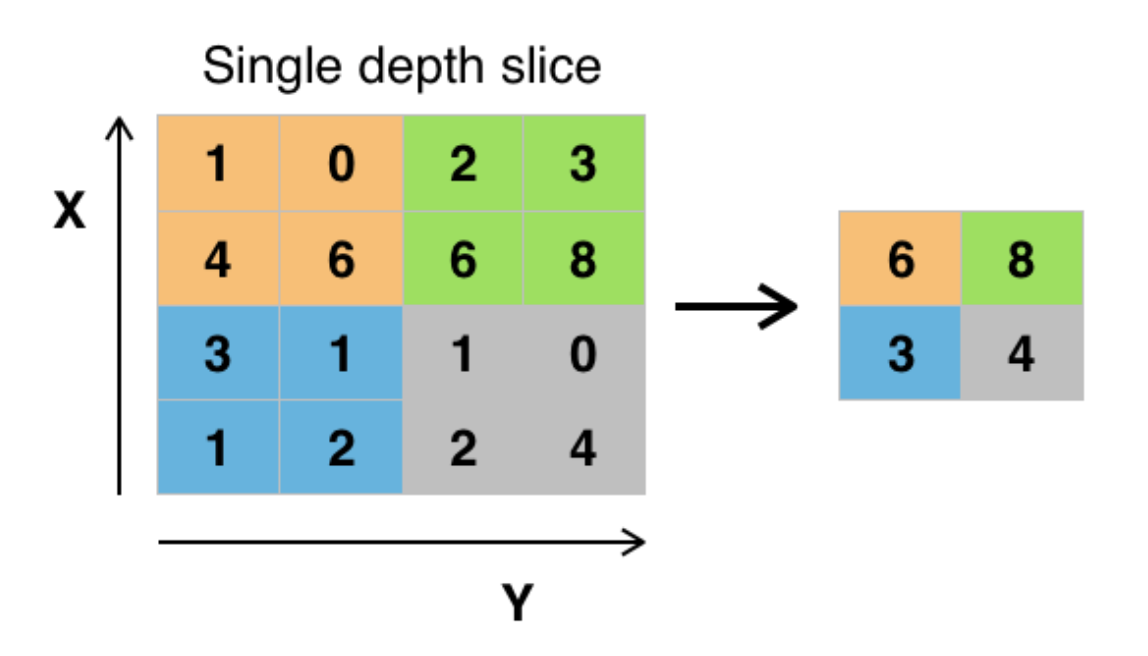daisy 633
dandelion 898
roses 641
sunflowers 699
tulips 799

# 迁移学习实践

## 文件结构

├── transfer_learning.py（运行代码）
├── flower_phtots
│   ├── daisy
│   ├── dandelion
│   ├── roses
│   └── ...
└── tensorflow_vgg
├── vgg16.py
├── vgg16.npy
└── ...


import os
import numpy as np
import tensorflow as tf

from tensorflow_vgg import vgg16
from tensorflow_vgg import utils


## 加载识花数据集

data_dir = 'flower_photos/'
contents = os.listdir(data_dir)
classes = [each for each in contents if os.path.isdir(data_dir + each)]


## 利用VGG16计算得到特征值

# 首先设置计算batch的值，如果运算平台的内存越大，这个值可以设置得越高
batch_size = 10
# 用codes_list来存储特征值
codes_list = []
# 用labels来存储花的类别
labels = []
# batch数组用来临时存储图片数据
batch = []

codes = None

with tf.Session() as sess:
# 构建VGG16模型对象
vgg = vgg16.Vgg16()
input_ = tf.placeholder(tf.float32, [None, 224, 224, 3])
with tf.name_scope("content_vgg"):
# 载入VGG16模型
vgg.build(input_)

# 对每个不同种类的花分别用VGG16计算特征值
for each in classes:
print("Starting {} images".format(each))
class_path = data_dir + each
files = os.listdir(class_path)
for ii, file in enumerate(files, 1):
# 载入图片并放入batch数组中
batch.append(img.reshape((1, 224, 224, 3)))
labels.append(each)

# 如果图片数量到了batch_size则开始具体的运算
if ii % batch_size == 0 or ii == len(files):
images = np.concatenate(batch)

feed_dict = {input_: images}
# 计算特征值
codes_batch = sess.run(vgg.relu6, feed_dict=feed_dict)

# 将结果放入到codes数组中
if codes is None:
codes = codes_batch
else:
codes = np.concatenate((codes, codes_batch))

# 清空数组准备下一个batch的计算
batch = []
print('{} images processed'.format(ii))


with open('codes', 'w') as f:
codes.tofile(f)

import csv
with open('labels', 'w') as f:
writer = csv.writer(f, delimiter='\n')
writer.writerow(labels)


## 准备训练集，验证集和测试集

from sklearn.preprocessing import LabelBinarizer

lb = LabelBinarizer()
lb.fit(labels)

labels_vecs = lb.transform(labels)


from sklearn.model_selection import StratifiedShuffleSplit

ss = StratifiedShuffleSplit(n_splits=1, test_size=0.2)

train_idx, val_idx = next(ss.split(codes, labels))

half_val_len = int(len(val_idx)/2)
val_idx, test_idx = val_idx[:half_val_len], val_idx[half_val_len:]

train_x, train_y = codes[train_idx], labels_vecs[train_idx]
val_x, val_y = codes[val_idx], labels_vecs[val_idx]
test_x, test_y = codes[test_idx], labels_vecs[test_idx]

print("Train shapes (x, y):", train_x.shape, train_y.shape)
print("Validation shapes (x, y):", val_x.shape, val_y.shape)
print("Test shapes (x, y):", test_x.shape, test_y.shape)


Train shapes (x, y): (2936, 4096) (2936, 5)
Validation shapes (x, y): (367, 4096) (367, 5)
Test shapes (x, y): (367, 4096) (367, 5)


## 训练网络

# 输入数据的维度
inputs_ = tf.placeholder(tf.float32, shape=[None, codes.shape])
# 标签数据的维度
labels_ = tf.placeholder(tf.int64, shape=[None, labels_vecs.shape])

# 加入一个256维的全连接的层
fc = tf.contrib.layers.fully_connected(inputs_, 256)

# 加入一个5维的全连接层
logits = tf.contrib.layers.fully_connected(fc, labels_vecs.shape, activation_fn=None)

# 计算cross entropy值
cross_entropy = tf.nn.softmax_cross_entropy_with_logits(labels=labels_, logits=logits)

# 计算损失函数
cost = tf.reduce_mean(cross_entropy)

# 得到最后的预测分布
predicted = tf.nn.softmax(logits)

# 计算准确度
correct_pred = tf.equal(tf.argmax(predicted, 1), tf.argmax(labels_, 1))
accuracy = tf.reduce_mean(tf.cast(correct_pred, tf.float32))


def get_batches(x, y, n_batches=10):
""" 这是一个生成器函数，按照n_batches的大小将数据划分了小块 """
batch_size = len(x)//n_batches

for ii in range(0, n_batches*batch_size, batch_size):
# 如果不是最后一个batch，那么这个batch中应该有batch_size个数据
if ii != (n_batches-1)*batch_size:
X, Y = x[ii: ii+batch_size], y[ii: ii+batch_size]
# 否则的话，那剩余的不够batch_size的数据都凑入到一个batch中
else:
X, Y = x[ii:], y[ii:]
# 生成器语法，返回X和Y
yield X, Y


# 运行多少轮次
epochs = 20
# 统计训练效果的频率
iteration = 0
# 保存模型的保存器
saver = tf.train.Saver()
with tf.Session() as sess:
sess.run(tf.global_variables_initializer())
for e in range(epochs):
for x, y in get_batches(train_x, train_y):
feed = {inputs_: x,
labels_: y}
# 训练模型
loss, _ = sess.run([cost, optimizer], feed_dict=feed)
print("Epoch: {}/{}".format(e+1, epochs),
"Iteration: {}".format(iteration),
"Training loss: {:.5f}".format(loss))
iteration += 1

if iteration % 5 == 0:
feed = {inputs_: val_x,
labels_: val_y}
val_acc = sess.run(accuracy, feed_dict=feed)
# 输出用验证机验证训练进度
print("Epoch: {}/{}".format(e, epochs),
"Iteration: {}".format(iteration),
"Validation Acc: {:.4f}".format(val_acc))
# 保存模型
saver.save(sess, "checkpoints/flowers.ckpt")


## 测试网络

with tf.Session() as sess:
saver.restore(sess, tf.train.latest_checkpoint('checkpoints'))

feed = {inputs_: test_x,
labels_: test_y}
test_acc = sess.run(accuracy, feed_dict=feed)
print("Test accuracy: {:.4f}".format(test_acc))